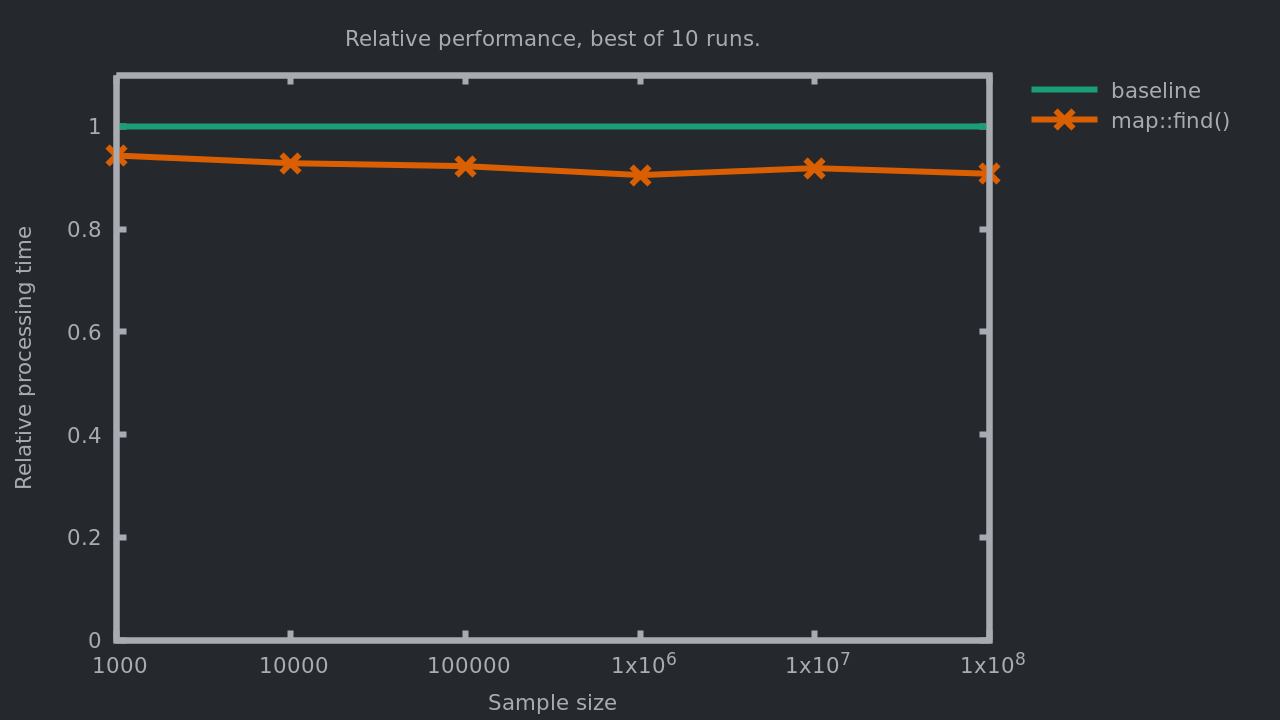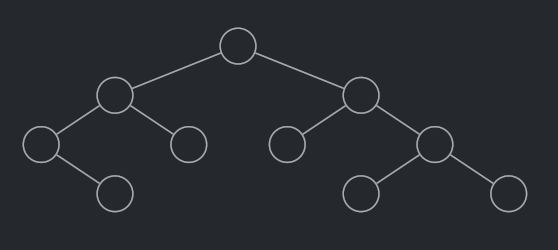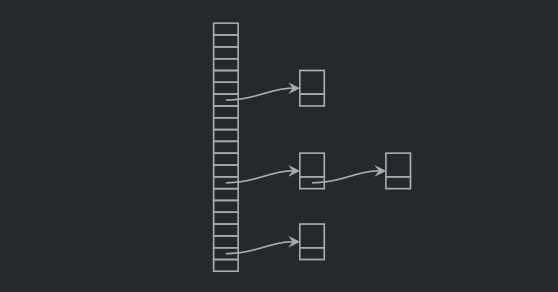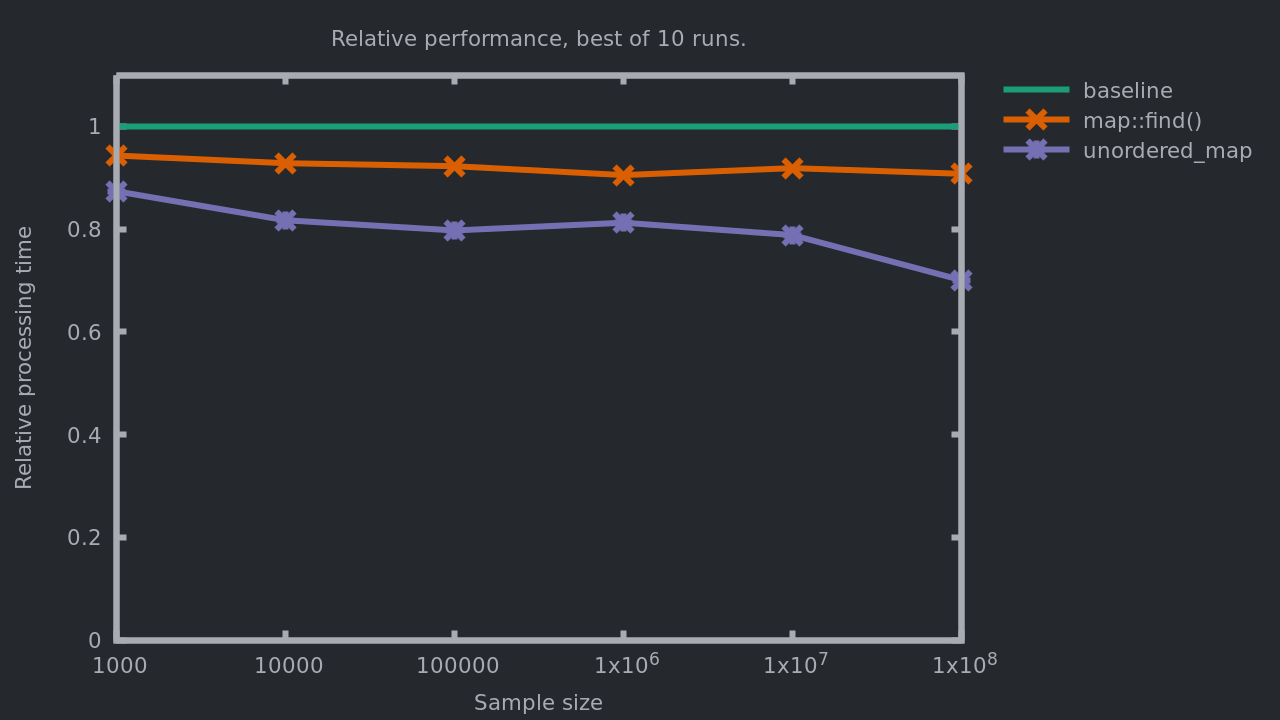Julien Jorge's Personal Website

## Effortless Performance Improvements in C++: std::unordered_map

Wed Mar 1, 2023

This is the second post in the series about effortless performance improvements in C++. Check the first post to get the full story!

Last time we observed that our hotspots were mostly located in the standard library, and we concluded that we may be using it wrong. The top offender being a function from `std::map`, let’s dig into that.

# Lookup in `std::map`

In our initial implementation we have a single map, used to store the key-value pairs obtained from the input. We are doing three lookups in this map. The first one is obvious: it is when we process a `"value"` record, where we need to check if the provided key has been set:

``````else if (tokens == "value")
{
// Obvious lookup in the map.
if (entries.find(tokens) != entries.end())
/* … */
``````

But there is also two less-obvious searches. Just one line below the previous scan:

``````else if (tokens == "value")
{
if (entries.find(tokens) != entries.end())
// Less obvious lookup in the map, via operator[].
result += "value:" + entries[tokens] + '\n';
``````

That’s right, calling `std::map::operator[]` does a lookup like `find` to check if there is already an entry with the given key. But since we did a search just above, couldn’t it be optimized by the compiler? Well, it’s complicated, but the answer is no.

Before showing the solution for this point, here is the third lookup, in the processing of a `"set"` record:

``````else if (tokens == "set")
// Another lookup in the map, via operator[]
entries[tokens] = tokens;
``````

Again, the call to `std::map::operator[]` does a lookup.

# Wasting less time with `std::map`

There is not so much we can do to avoid the search in the processing of a `"set"` record. We could use `std::map::emplace` to save the overwritting of an existing key, but it would change the behavior of our program. So we are going to leave it as it is.

In the processing of `"value"` we could abuse the fact that accessing a non-existent key via `std::map::operator[]` creates a new value using the default constructor of its type. In our case it would be an empty string, so the output would be the same:

``````else if (tokens == "value")
{
// entries[tokens] is the existing value if any, or an empty
// string if none.
result += "value:" + entries[tokens] + '\n';
``````

But by doing so we would insert a new entry in the map for each non-existent key we test! This is not what we want.

The proper solution to get an optional value associated with the key is to keep the iterator returned by `std::map::find()`:

``````else if (tokens == "value")
{
// Single search in the map
const entry_map::const_iterator it = entries.find(tokens);

if (it != entries.end())
// Here we can access the value via the iterator.
result += "value:" + it->second + '\n';
else
result += "value:\n";

++line_count;
}
``````

Just like in the initial code, we search the map using `std::map::find`, but now we store the result in a local variable. The result is an iterator that we can compare with `entries.end()`, as before. Now, if the iterator is not the end iterator, then we can get the value from the map by dereferencing the iterator and accessing its `second` member.

This is not a huge change, what difference can it make?Ouch! 5 to 9% faster with such a small change! Quite good isn’t it? Let’s check again the hotspots with `perf`:

• 26% in `std::map<std::string, std::string>::find`.
• Under which the top offender is `std::string::operator<`.
• 15% in `tokenize`.
• Mostly in `std::vector<std::string>::push_back`.
• 10% in `std::map<std::string, std::string>::operator[]`.
• Again with `std::string::operator<` as the top offender.
• 6% in `std::getline`.
• 5% in `replace`.
• With `std::string::find` as the top offender.

The time spent in `std::map::operator[]` went down from 15% to 10%. This is now less of a problem than the call to `tokenize` but we still have `std::map::find` at the top. As we saw before, there’s not so much we can do to avoid these calls, so let’s see if there would be different approach as a more efficient replacement.

# What’s an `std::map` anyway?

The implementation of `std::map` is a binary tree, where each node points to two sub-trees: one where the items are smaller than the one in the current node, and one where the items are greater. Conceptually, it looks like this:The algorithmic complexity for searching, inserting, or removing an entry is O(log2(n)), where n is the number of items in the container.

Since C++11 the standard library provides another associative table in the form of `std::unordered_map`. Its implementation is a hash table: an integral hash is computed for each item, then this hash is used as an index in a table where the items are stored. If many items end up in the same slot, they are chained, as in a linked list. Conceptually, it looks like this:The algorithmic complexity for searching, inserting, or removing an entry is O(1), which is clearly better than the one of `std::map`.

Hidden in the cost of `std::map<std::string, T>` is the comparison of the strings as we scan the map. On the other hand, `std::hash_map<std::string, T>` has to compute a hash of the provided strings.

# Switching to `std::unordered_map`

Let’s apply the following changes in our program and see how it impacts its performance.

`````` -5,5 +5,5
#include "tokenize.hpp"

-#include <map>
+#include <unordered_map>
#include <sstream>

-27,5 +27,5
std::string result;
std::uint64_t next_date = 0;
- std::map<std::string, std::string> entries;
+ std::unordered_map<std::string, std::string> entries;

while (std::getline(iss, line))
``````

Very easy modification. What difference does it make?Up to 30% improvements over the baseline, and 23% compared with the previous implementation! Note that it does not matter if the map is small or large, it is always a win to use `std::unordered_map` here.

Let’s look at the `perf` profile.

• 17% in `std::unordered_map<std::string, std::string>::operator[]`.
• 17% in `tokenize`.
• Mostly in `std::vector<std::string>::push_back`.
• 12% in `std::unordered_map<std::string, std::string>::find`.
• 7% in `replace`.
• With `std::string::find` as the top offender.
• 6% in `std::getline`.

Looks like our maps are less of a problem now, and `tokenize` is going up with its uses of `std::vector`. Let’s see if we can do something about it in part 3.